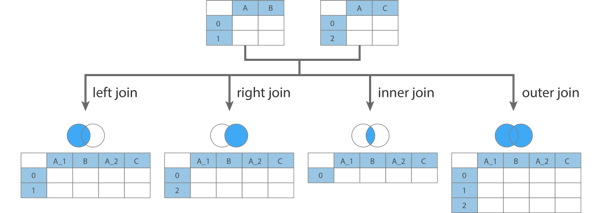a shot of dev knowledge

RELATED TAGS

# Three ways to combine DataFrames in Pandas

## Pandas join() function

This function allows the lowest level of control. It will join the rows from the two tables based on a common column or index. Have a look at the illustration below to understand various type of joins.Type of join operations

Now, let’s have a look at the coding part.

df1 = pd.DataFrame({'key': ['K0', 'K1', 'K2', 'K3', 'K4', 'K5'],
'A': ['A0', 'A1', 'A2', 'A3', 'A4', 'A5']})

df2 = pd.DataFrame({'key': ['K0', 'K1', 'K2'],
'B': ['B0', 'B1', 'B2']})

print(df1)
print(df2)
Defining two sample DataFrames

The output of the above code snippet is:

  key   A
0  K0  A0
1  K1  A1
2  K2  A2
3  K3  A3
4  K4  A4
5  K5  A5
key   B
0  K0  B0
1  K1  B1
2  K2  B2


Now, let’s join the two DataFrames.

df1.join(df2, lsuffix='_caller', rsuffix='_other')

The output of the above join operation will be:

  key_caller   A key_other    B
0         K0  A0        K0   B0
1         K1  A1        K1   B1
2         K2  A2        K2   B2
3         K3  A3       NaN  NaN
4         K4  A4       NaN  NaN
5         K5  A5       NaN  NaN


Explanation :

• By default, join() does a left join, but you can change the type of join by providing a value for the how parameter in the join() function as how='type_of_join'
• The parameterlsuffix is the suffix that will be added to the column name from the left frame’s overlapping columns.
• The parameter rsuffix is the suffix that will be added to the column name from the right frame’s overlapping columns.

## Pandas merge() function

This function is also used to combine or join two DataFrames with the same columns or indices. More or less, it does the same thing as join().

However, merge() allows us to specify what columns to join on for both the left and right DataFrames.

merge() is useful when we don’t want to join on the index.

df1 = pd.DataFrame({'lkey': ['foo', 'bar', 'baz', 'foo'],
'value': [1, 2, 3, 5]})
df2 = pd.DataFrame({'rkey': ['foo', 'bar', 'baz', 'foo'],
'value': [5, 6, 7, 8]})

print(df1)
print(df2)
Defining two sample DataFrames

The output of the above code is:

    lkey value
0   foo      1
1   bar      2
2   baz      3
3   foo      5
rkey value
0   foo      5
1   bar      6
2   baz      7
3   foo      8


Now, let’s merge the two DataFrames.

df1.merge(df2, left_on='lkey', right_on='rkey')

The output of the above code is:

  lkey  value_x rkey  value_y
0  foo        1  foo        5
1  foo        1  foo        8
2  foo        5  foo        5
3  foo        5  foo        8
4  bar        2  bar        6
5  baz        3  baz        7


Explanation:

• The parameter left_on is the column or index level names to join on in the left DataFrame.
• The parameter right_on is the column or index level names to join on in the right DataFrame.
• By default, the merge() function performs an inner join, but you can change it by passing the parameter value how='type_of_join'.

## Pandas concat() function

This function is used to append one (or more) DataFrames stacked below the other (or sideways, depending on whether the axis option is set to 0 or 1).

Also, make sure that the dimensions of the DataFrames should match along the axis while concatenating.

df1 = pd.DataFrame({'Key': ['b', 'b', 'a', 'c', 'a', 'a', 'b'],
'data1': range(7)})

df2 = pd.DataFrame({'Key': ['a', 'b', 'd'],
'data2': range(3)})

print(df1)
print(df2)

The output of the above code is:

   Key  data1
0   b   0
1   b   1
2   a   2
3   c   3
4   a   4
5   a   5
6   b   6
Key data2
0   a   0
1   b   1
2   d   2


Now, let’s concatenate the DataFrames.

pd.concat([df1, df2])

The output of the above code is:

   Key data1 data2
0   b   0     NaN
1   b   1     NaN
2   a   2     NaN
3   c   3     NaN
4   a   4     NaN
5   a   5     NaN
6   b   6     NaN
0   a   NaN   0
1   b   NaN   1
2   d   NaN   2


Explanation:

• The dataframe df2 is appended after df1.
• NaN values denote that the values for that column are not present in the DataFrame.

## Which to use and when to use?

1. The join() method works best when we are joining DataFrames on their indexes.
2. The merge() method is more versatile and allows us to specify columns, besides the index to join on, for both DataFrames.
3. We cannot use concat() if our DataFrames’ dimensions do not match along the axis in which we are trying to concatenate.
4. The concat() has inner (default) and outer joins only, whereas merge() has left, right, outer, and inner (default) joins.

RELATED TAGS

RELATED COURSES

View all Courses

Keep Exploring

Learn in-demand tech skills in half the time